# Rajasthan Board Question Papers for Class 12th Maths 2016 With Solutions In PDF

Rajasthan Class 12 Maths 2016 question paper with solutions are provided here in a downloadable pdf format and also in the text, so that the students can obtain appropriate and accurate solutions for all the questions. Along with the solutions, Maths question paper 2016 Class 12 is also available here for reference. Students are able to access all the Rajasthan board previous year Maths question papers.

## Rajasthan Board Class 12 Maths Previous Year Paper 2016 with Solutions

Solving these RBSE Class 12 Maths Previous Year Question Paper 2016 and then referring to the solutions will help the students to gauge their performance and exam preparations. The solutions provided by BYJU’S will assist the students in their preparation and also will give them an idea of the difficulty level of the questions that can be asked in the upcoming board exams. Solving the question papers will make the students understand the pattern followed by the examiners, while setting the paper of board exams.

### QUESTION PAPER CODE 412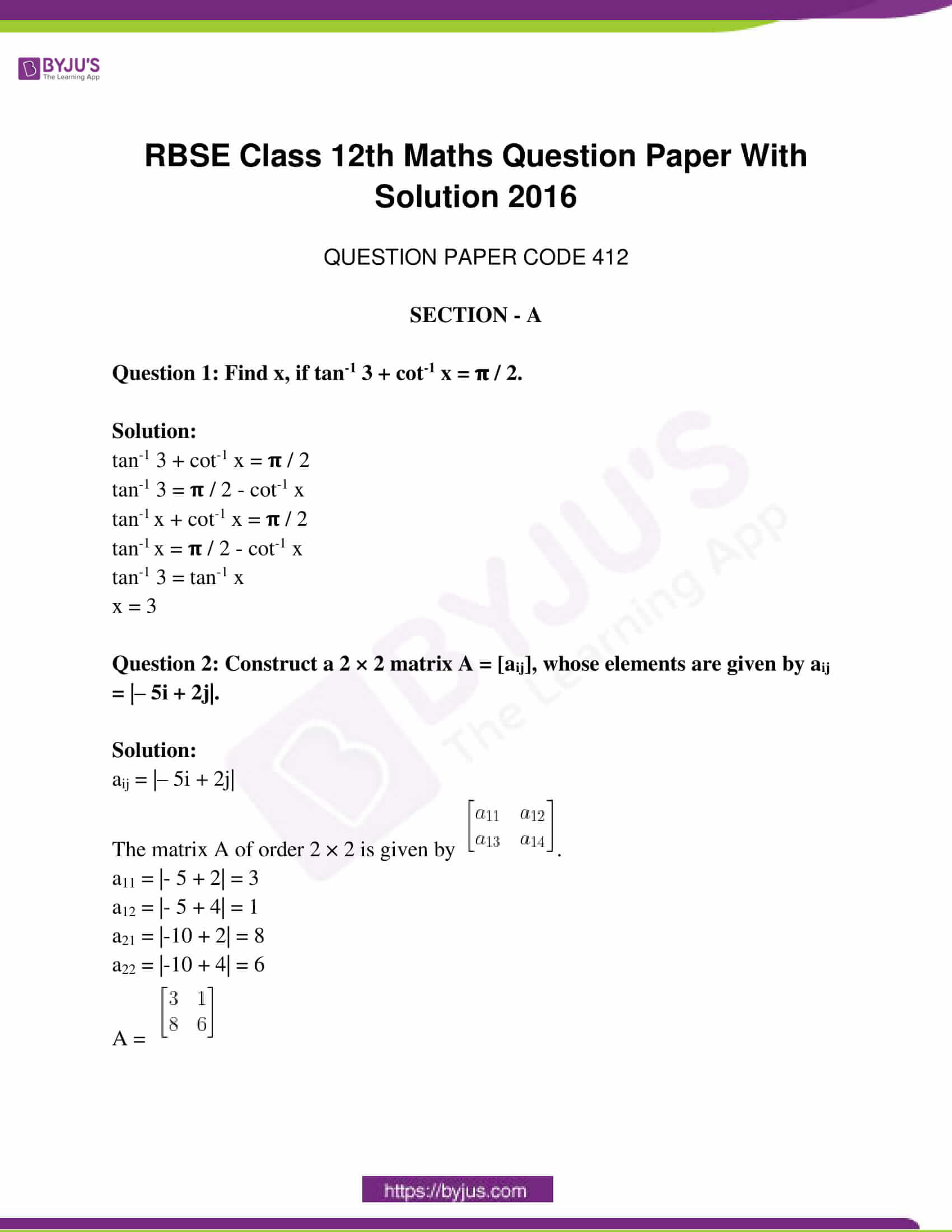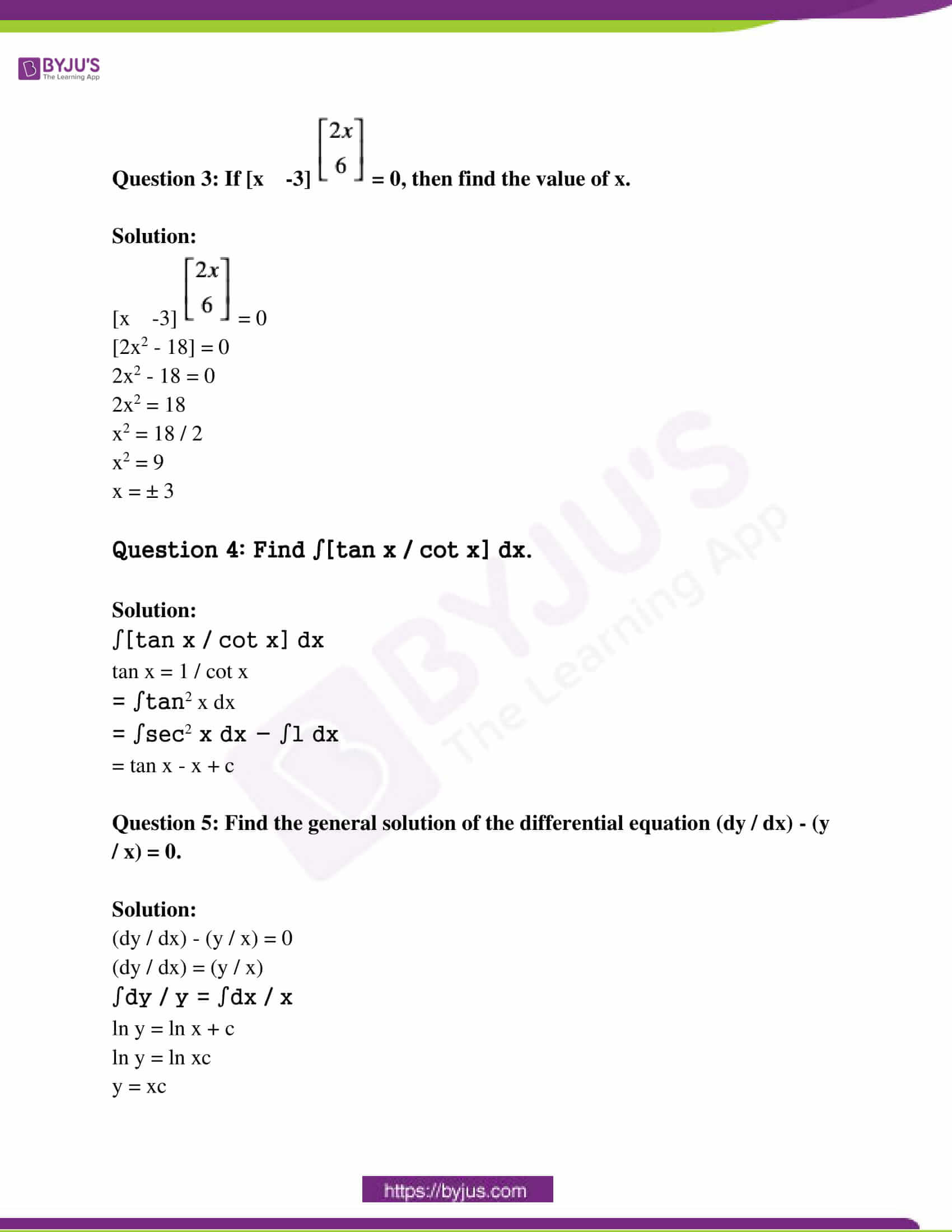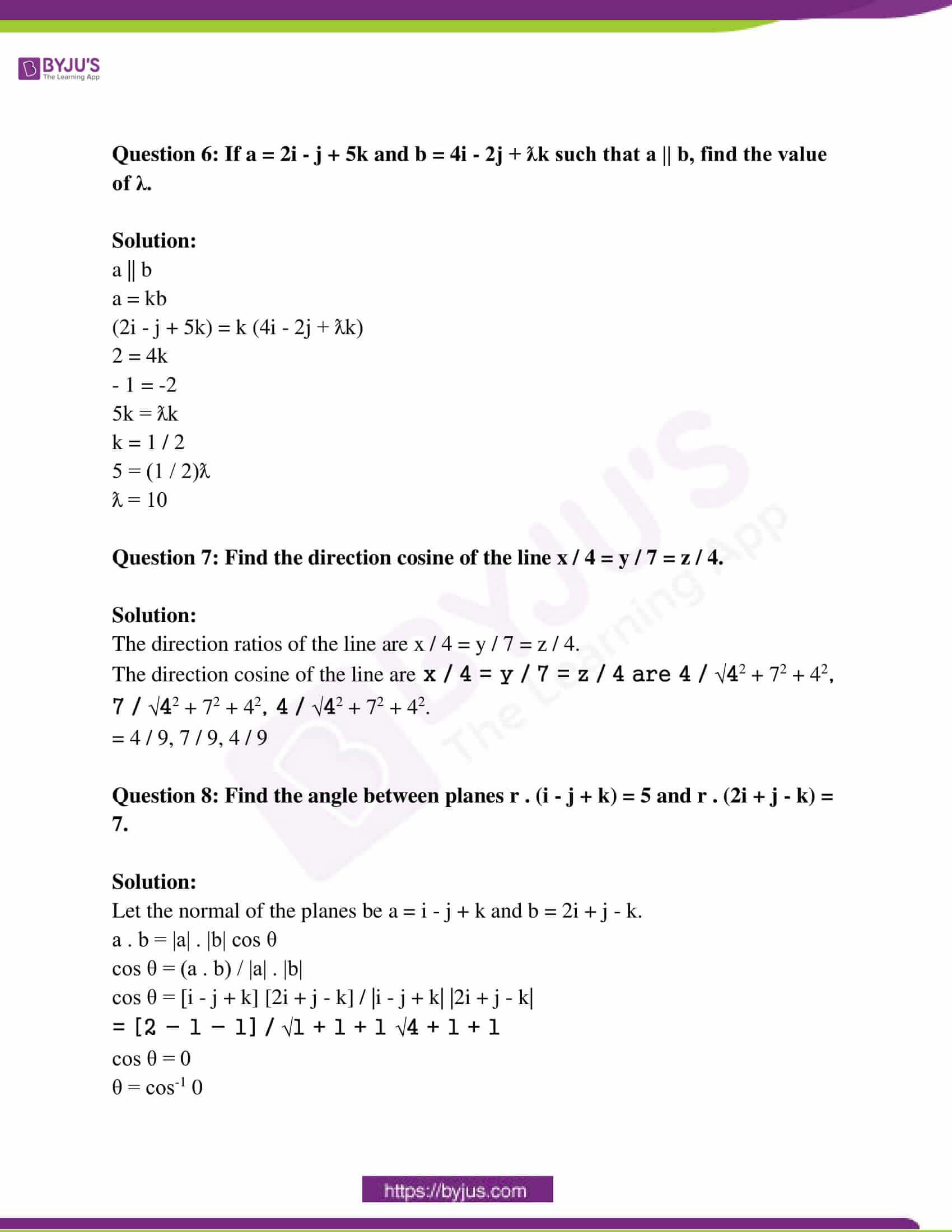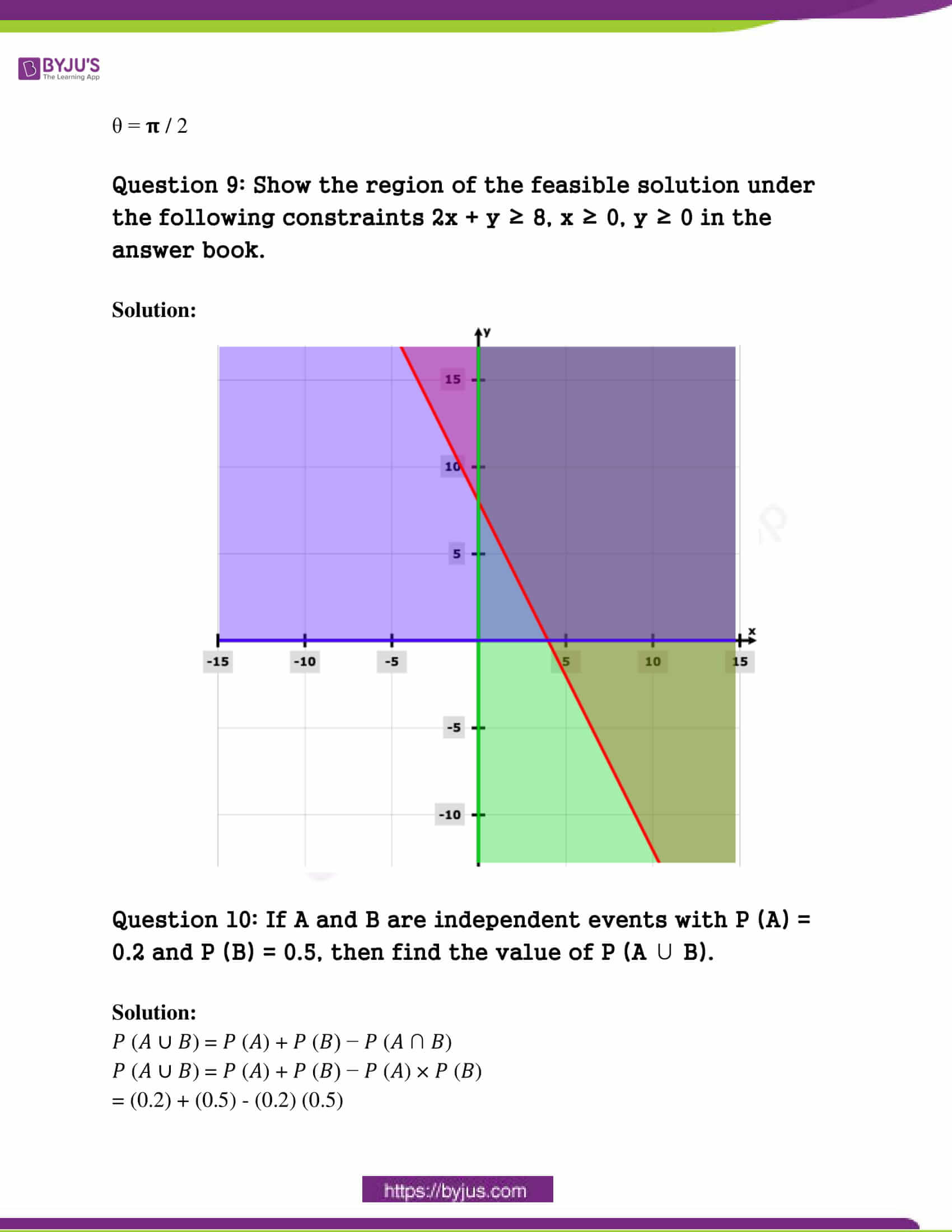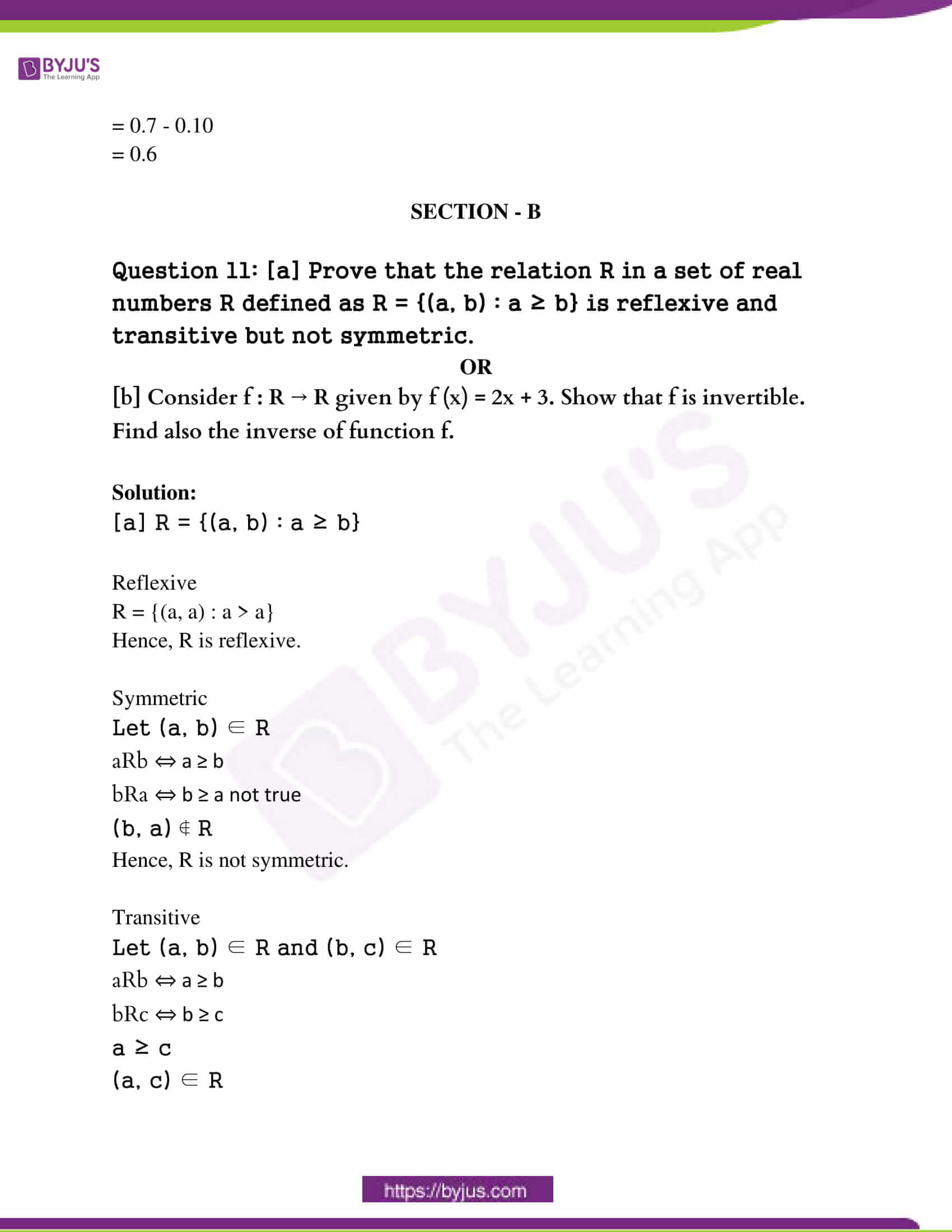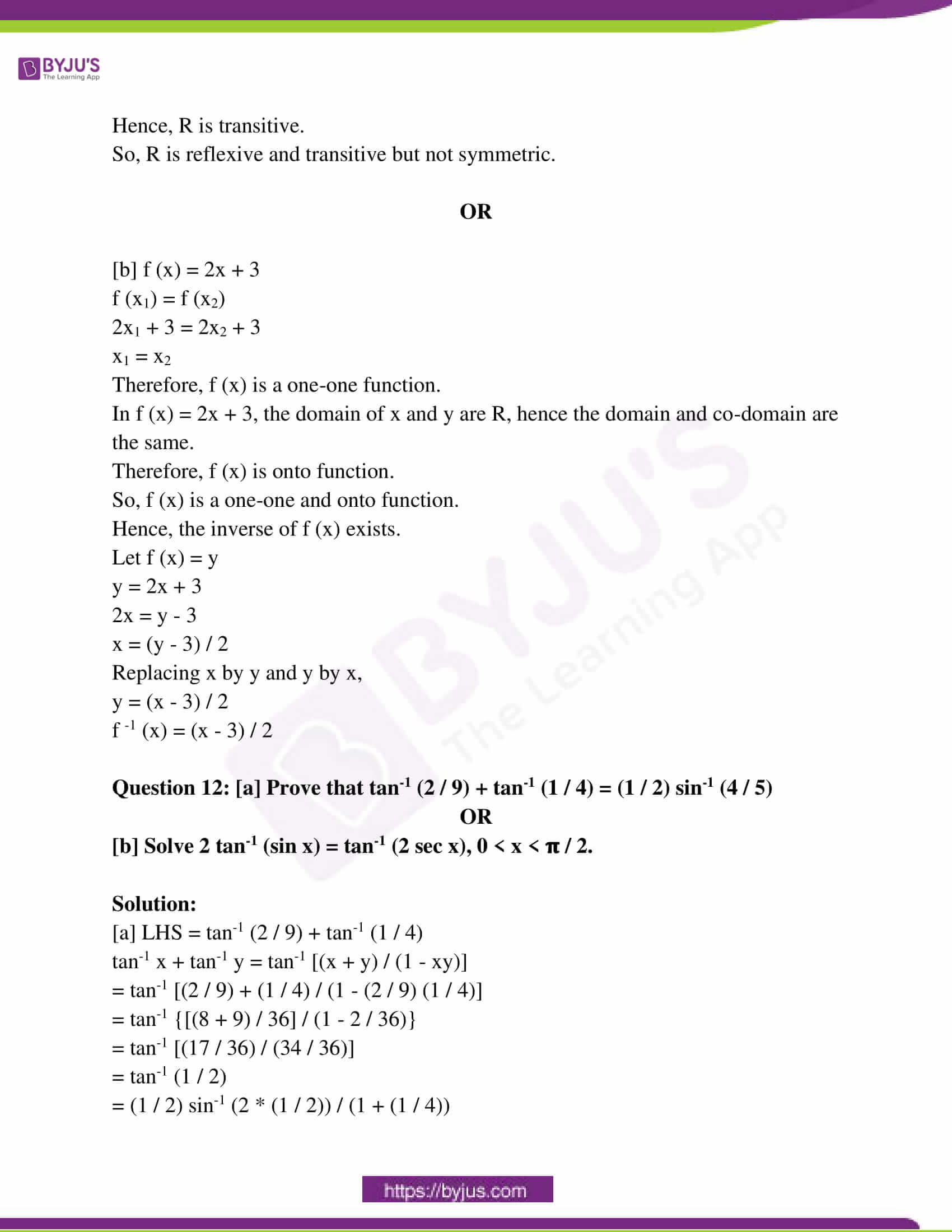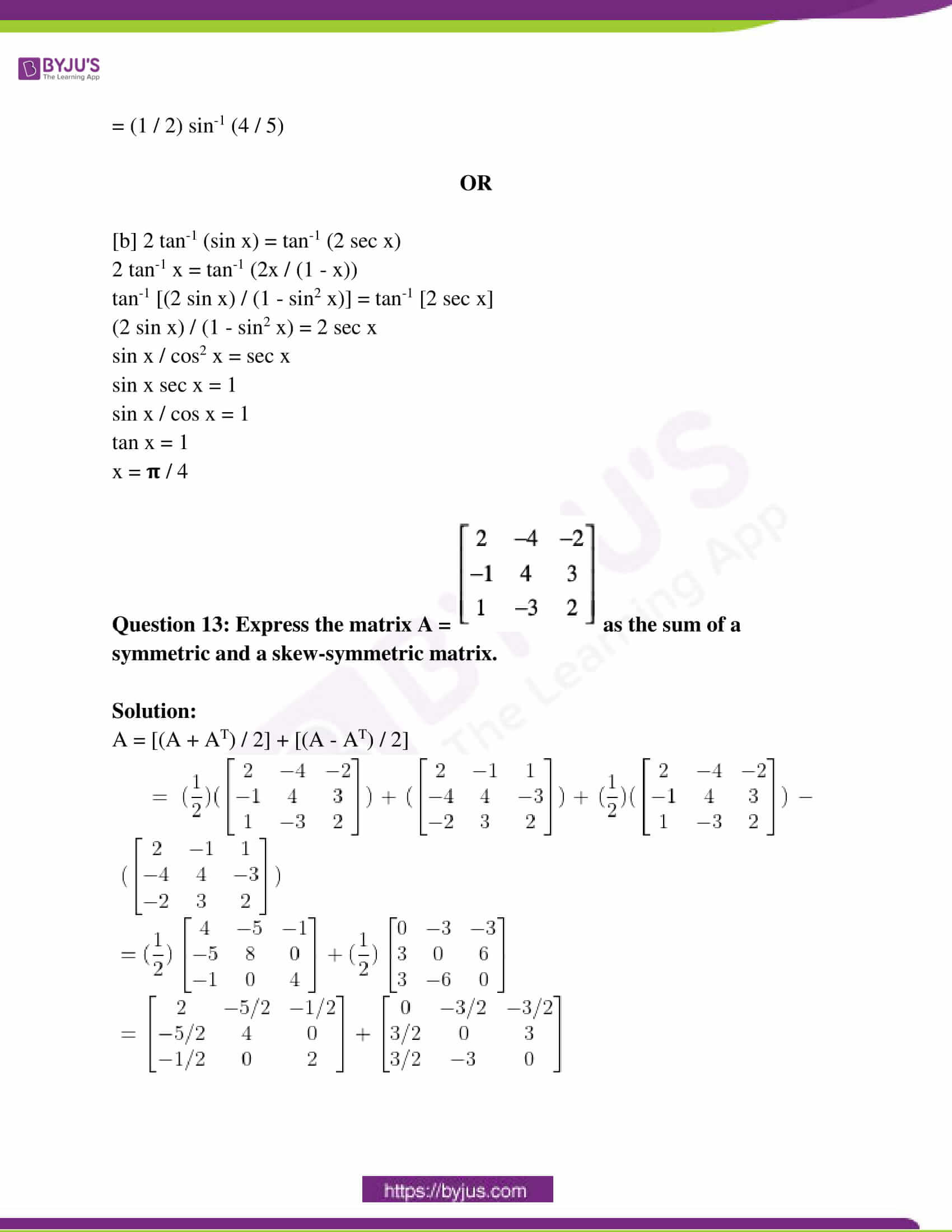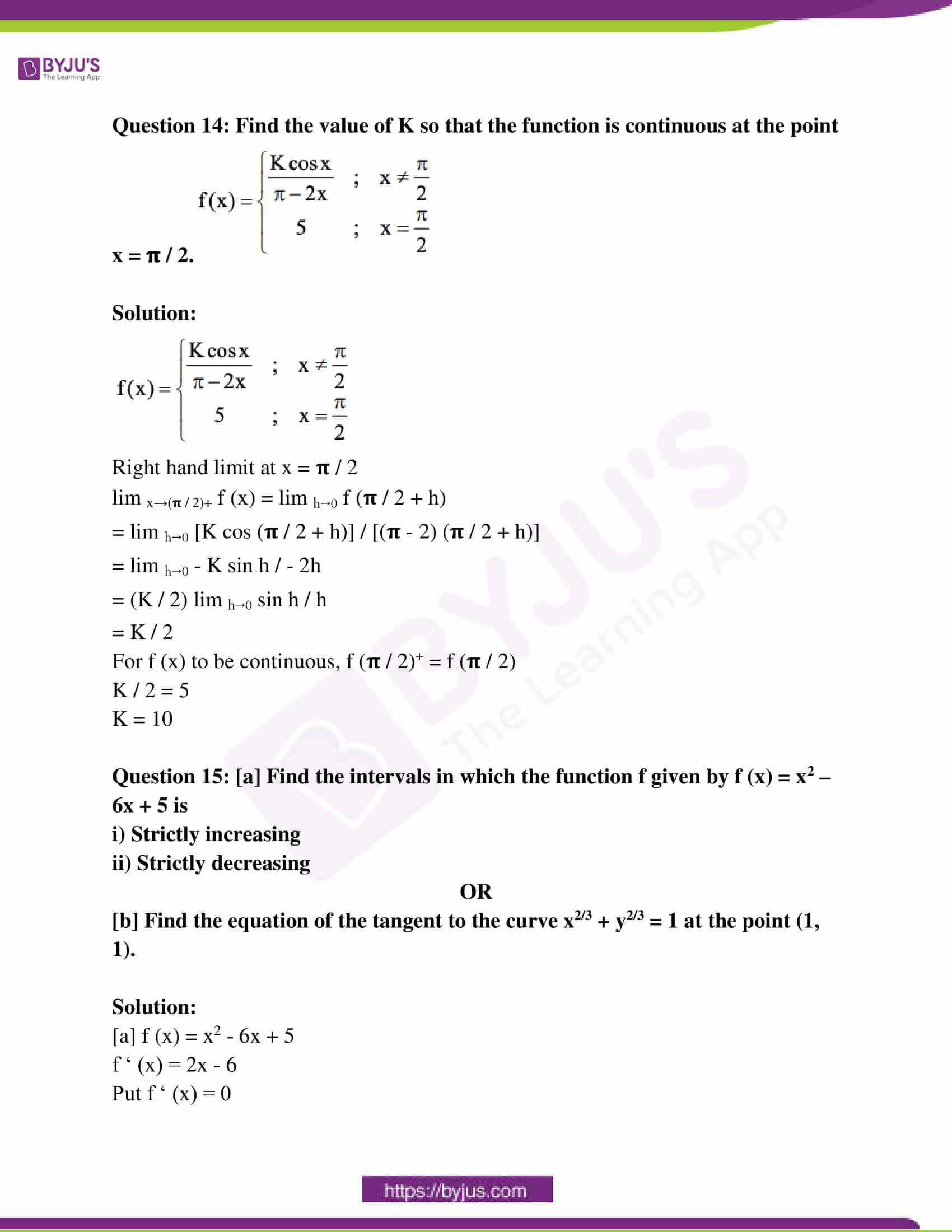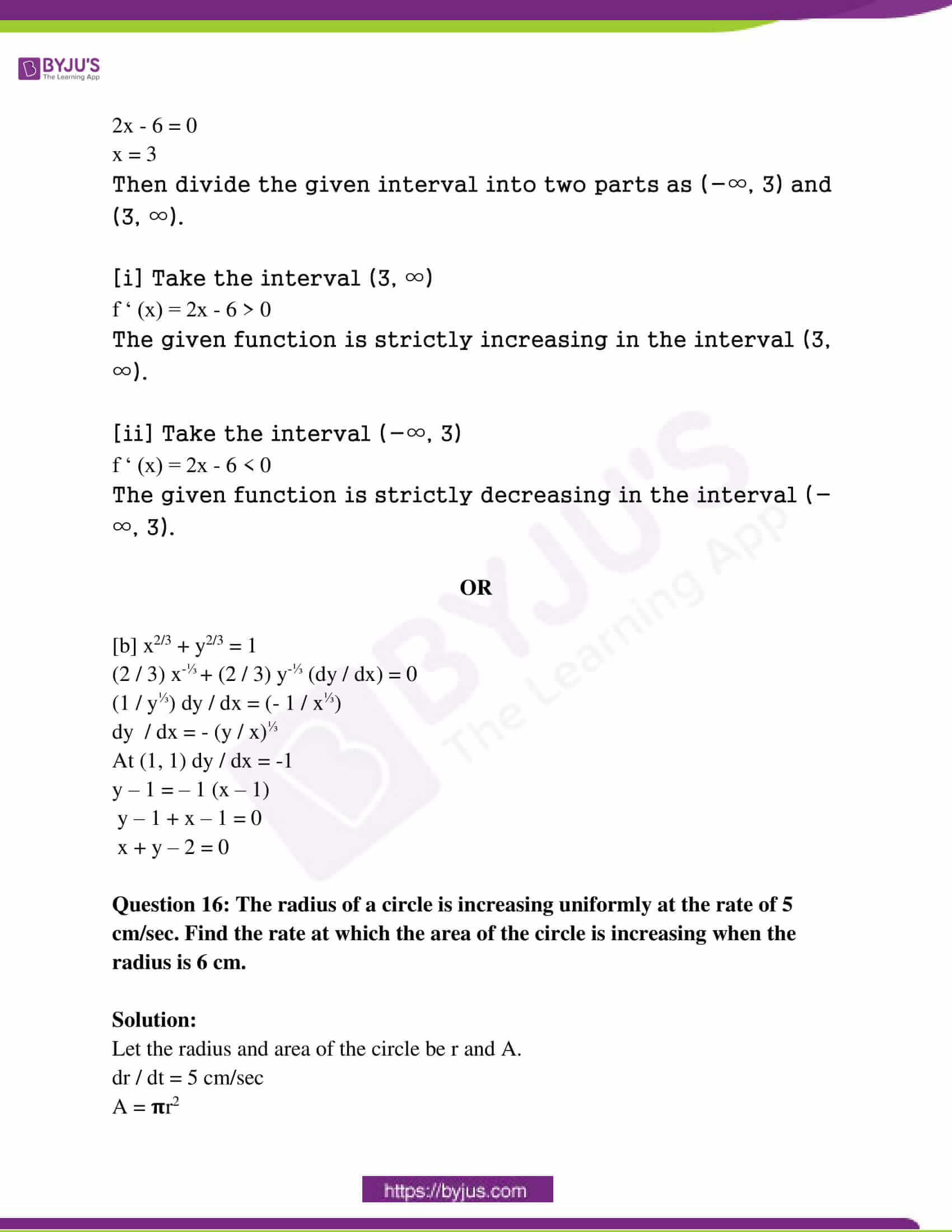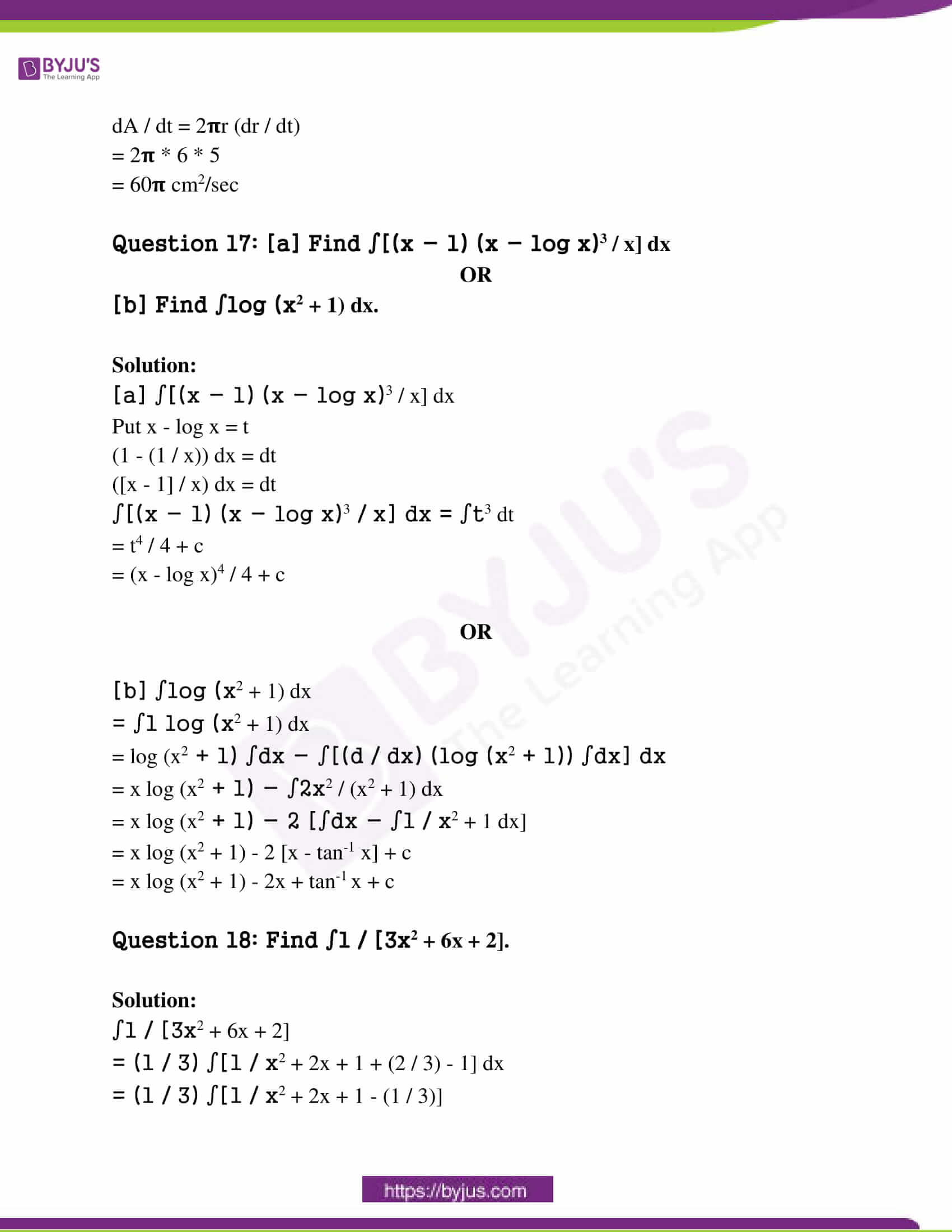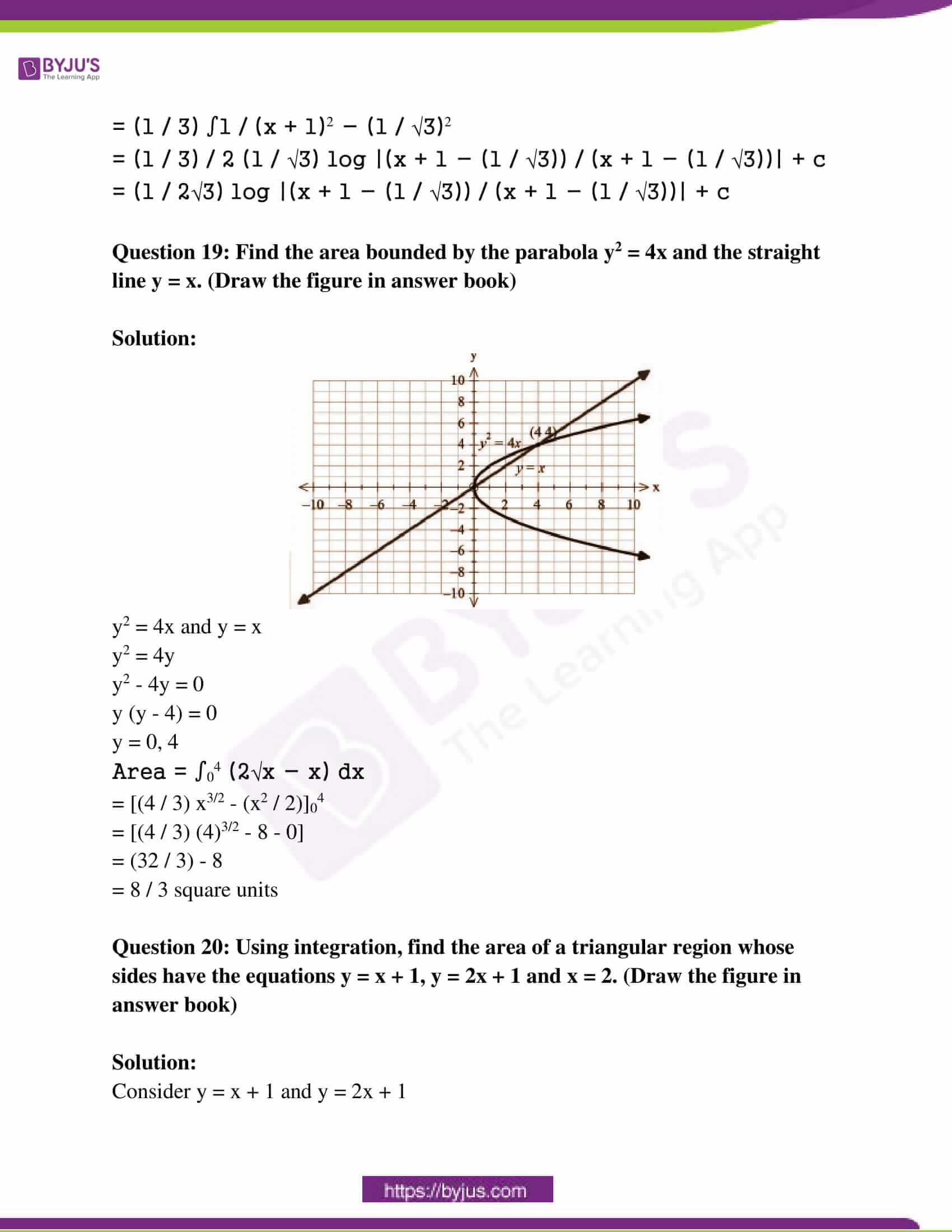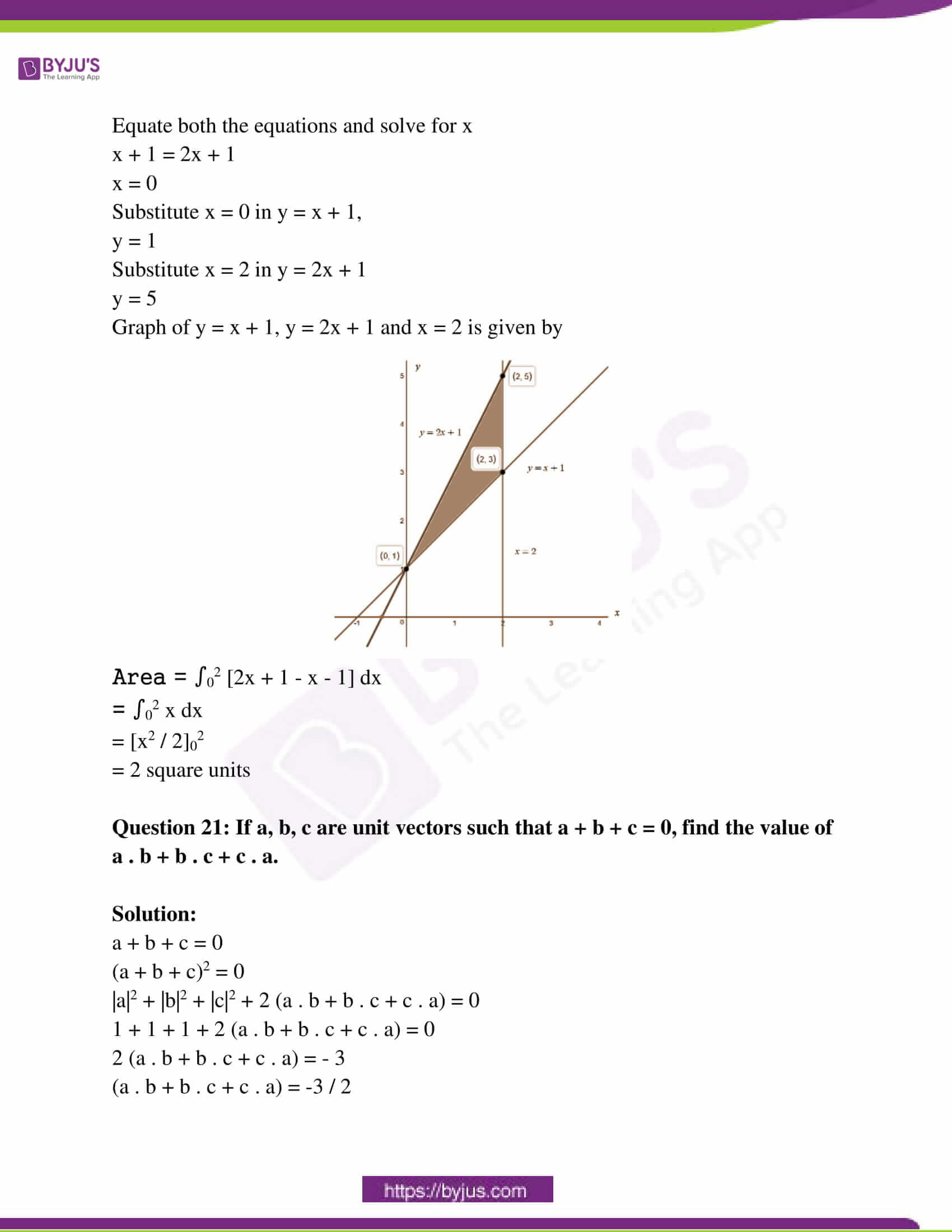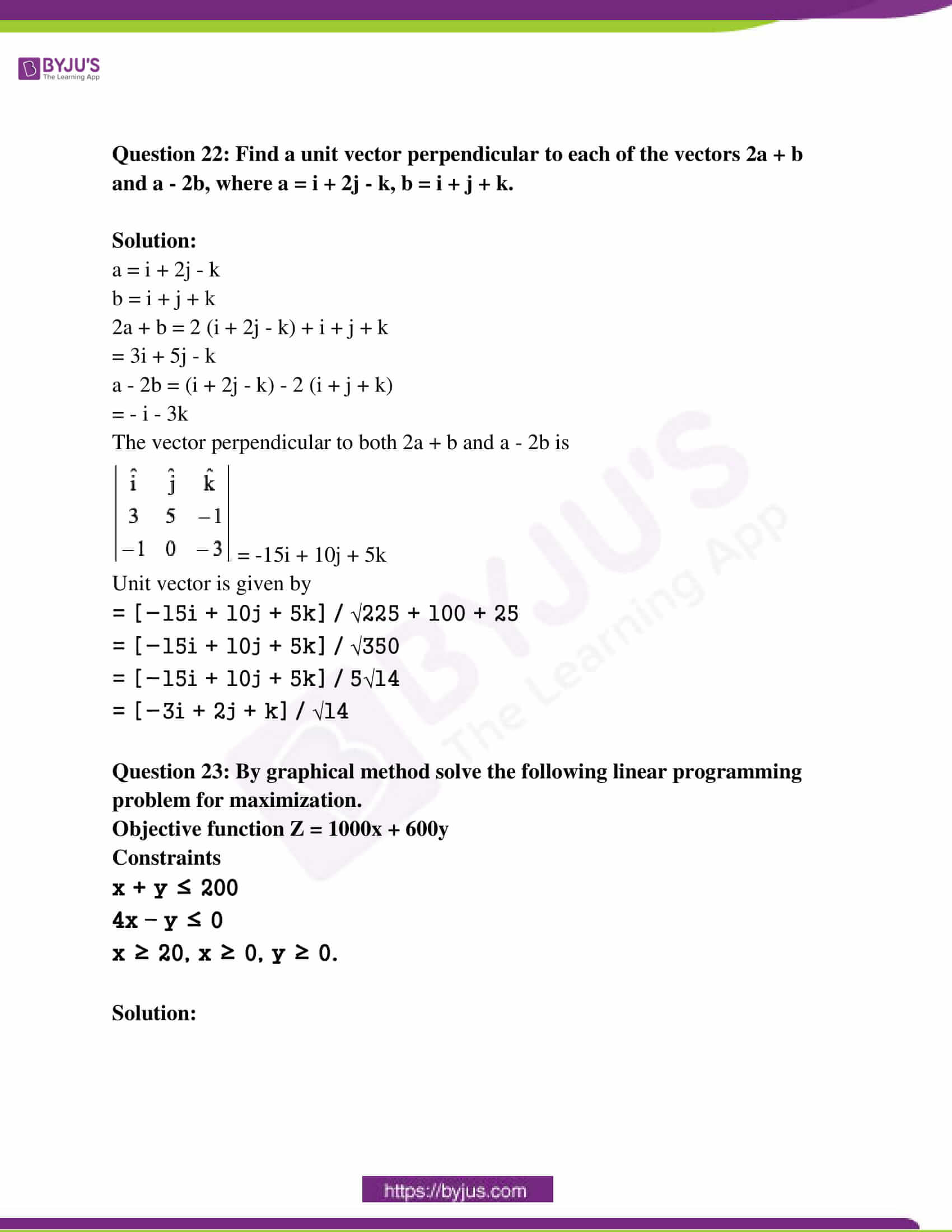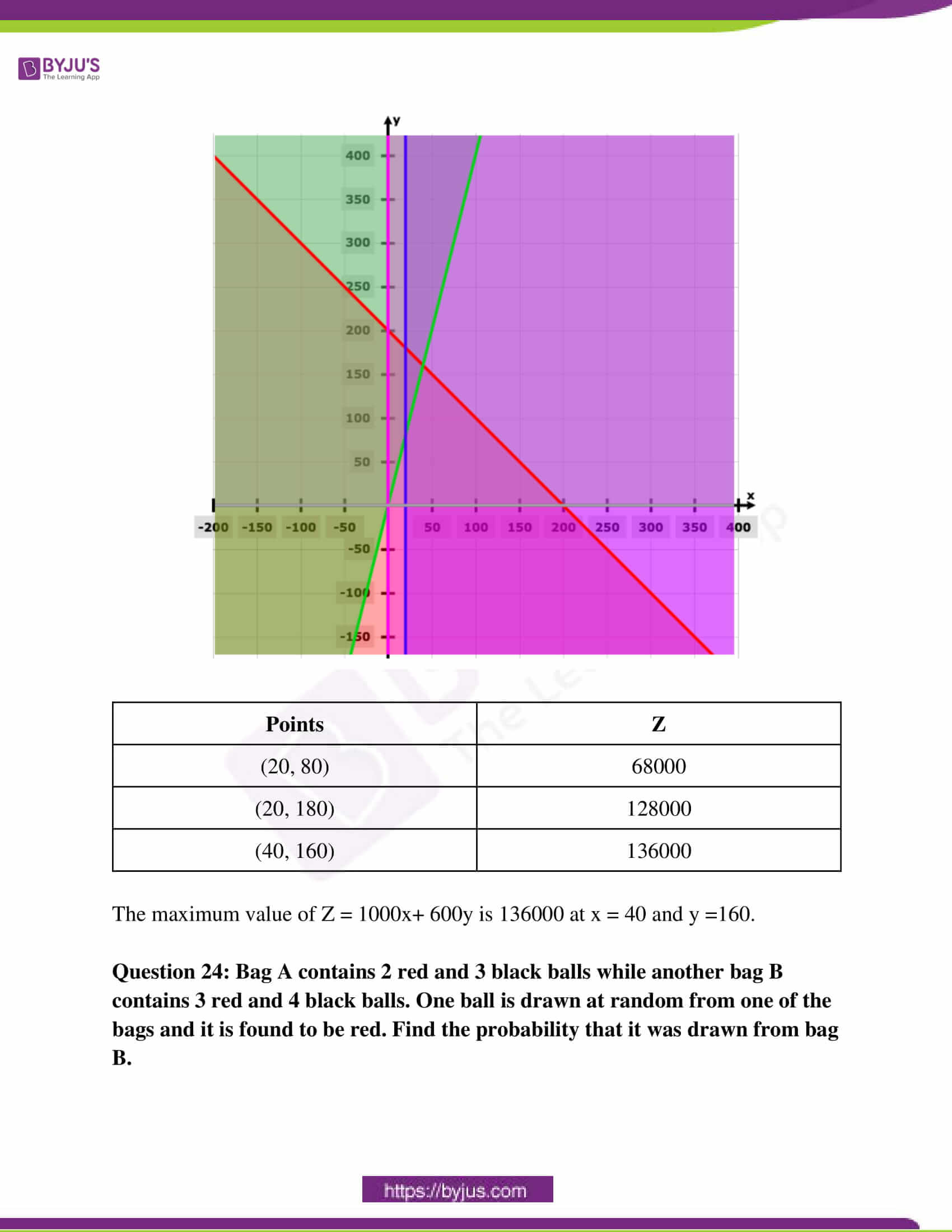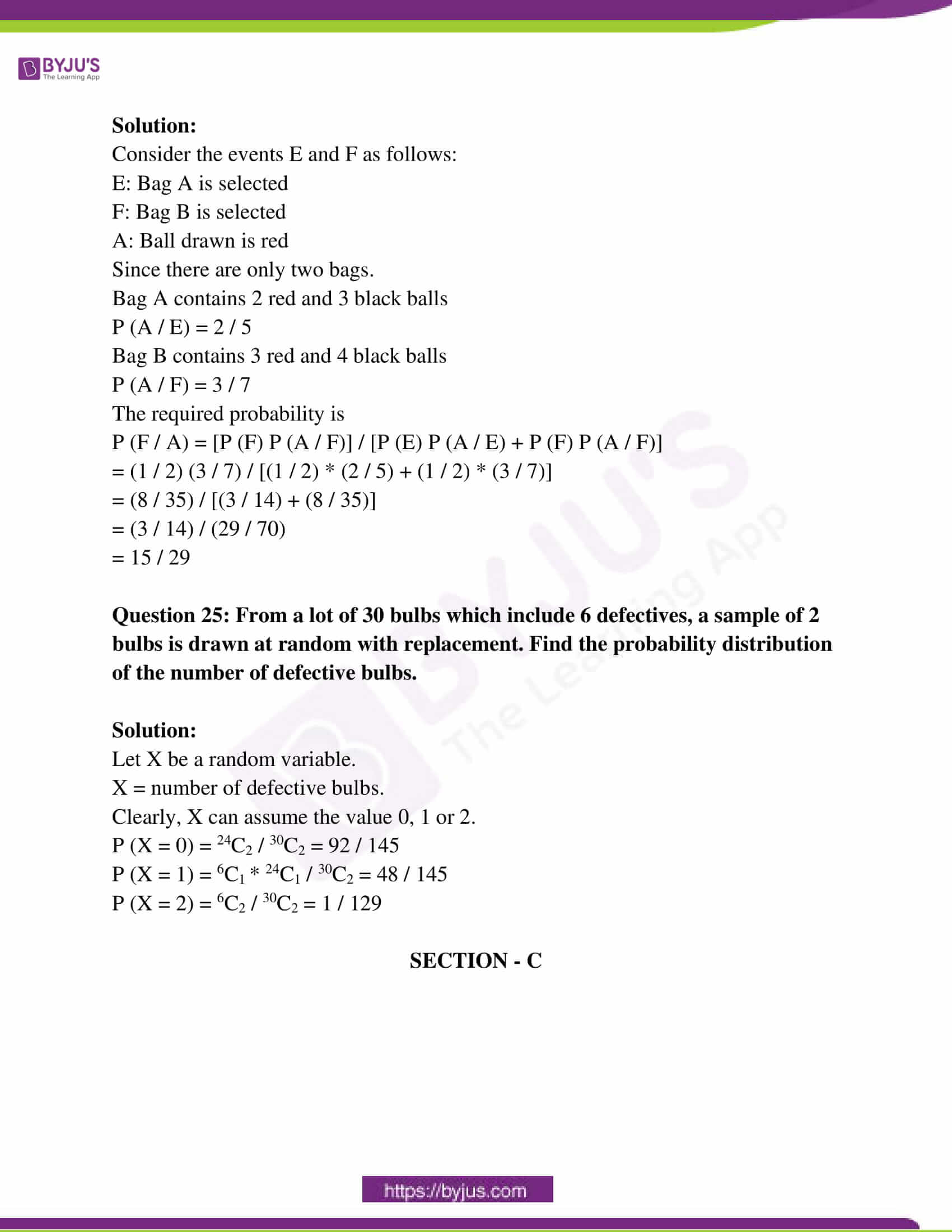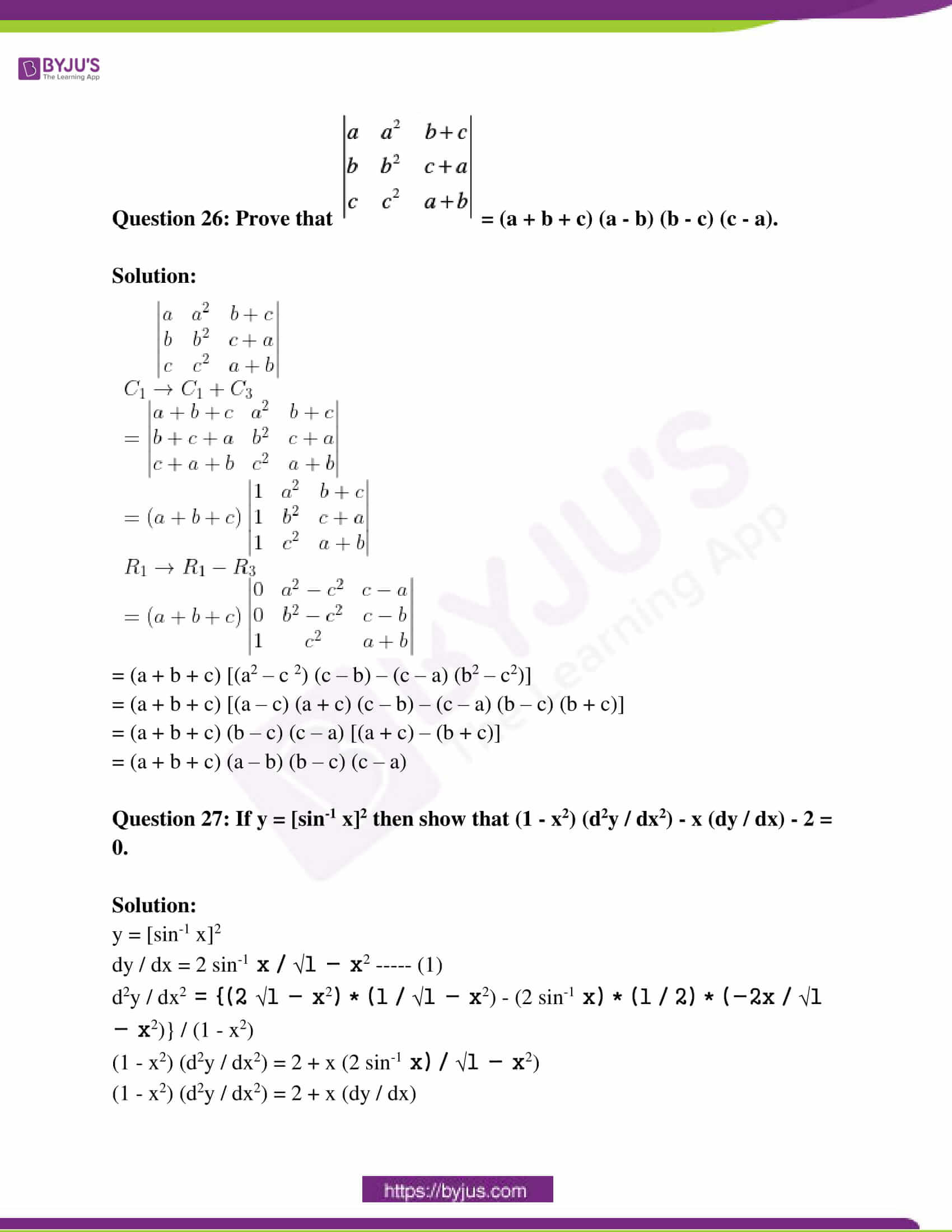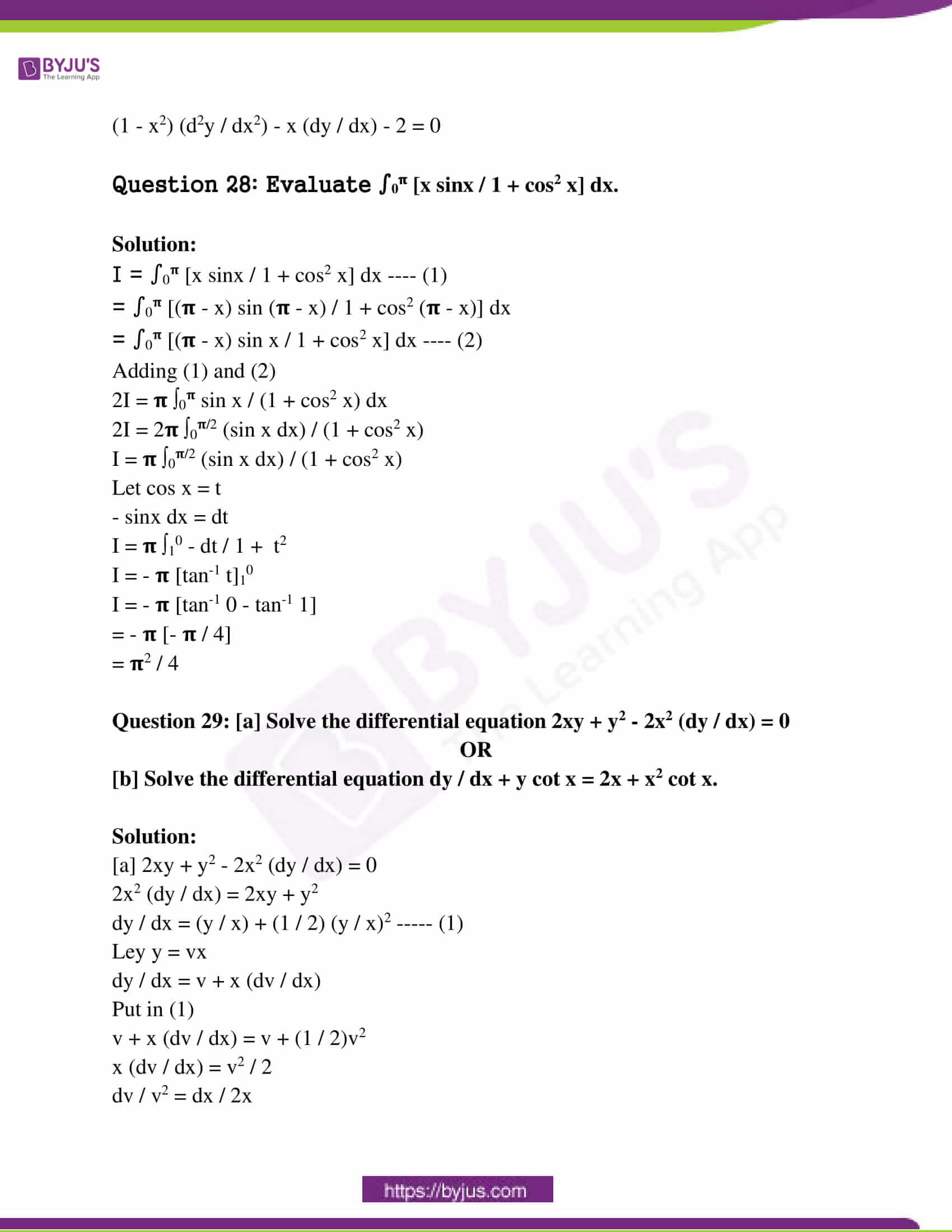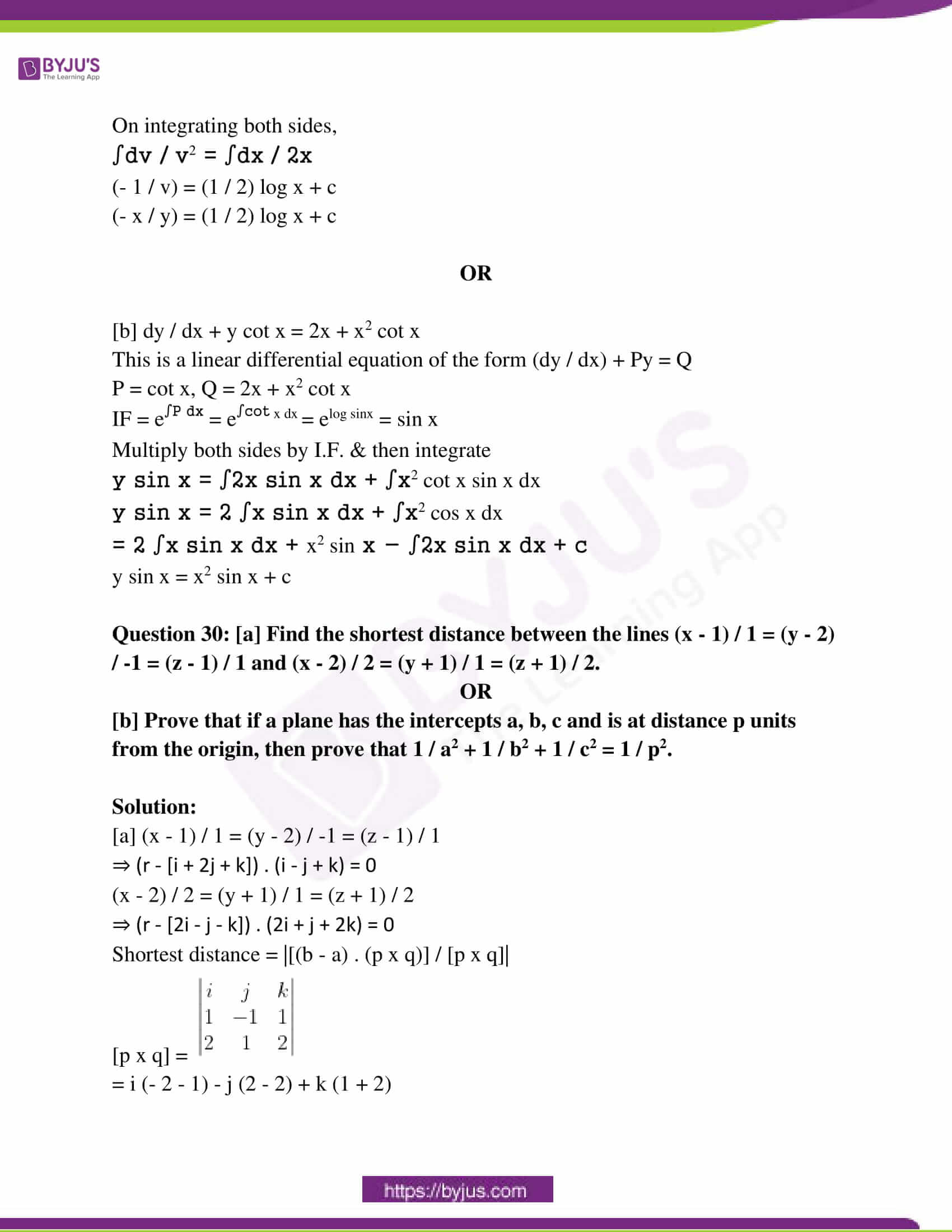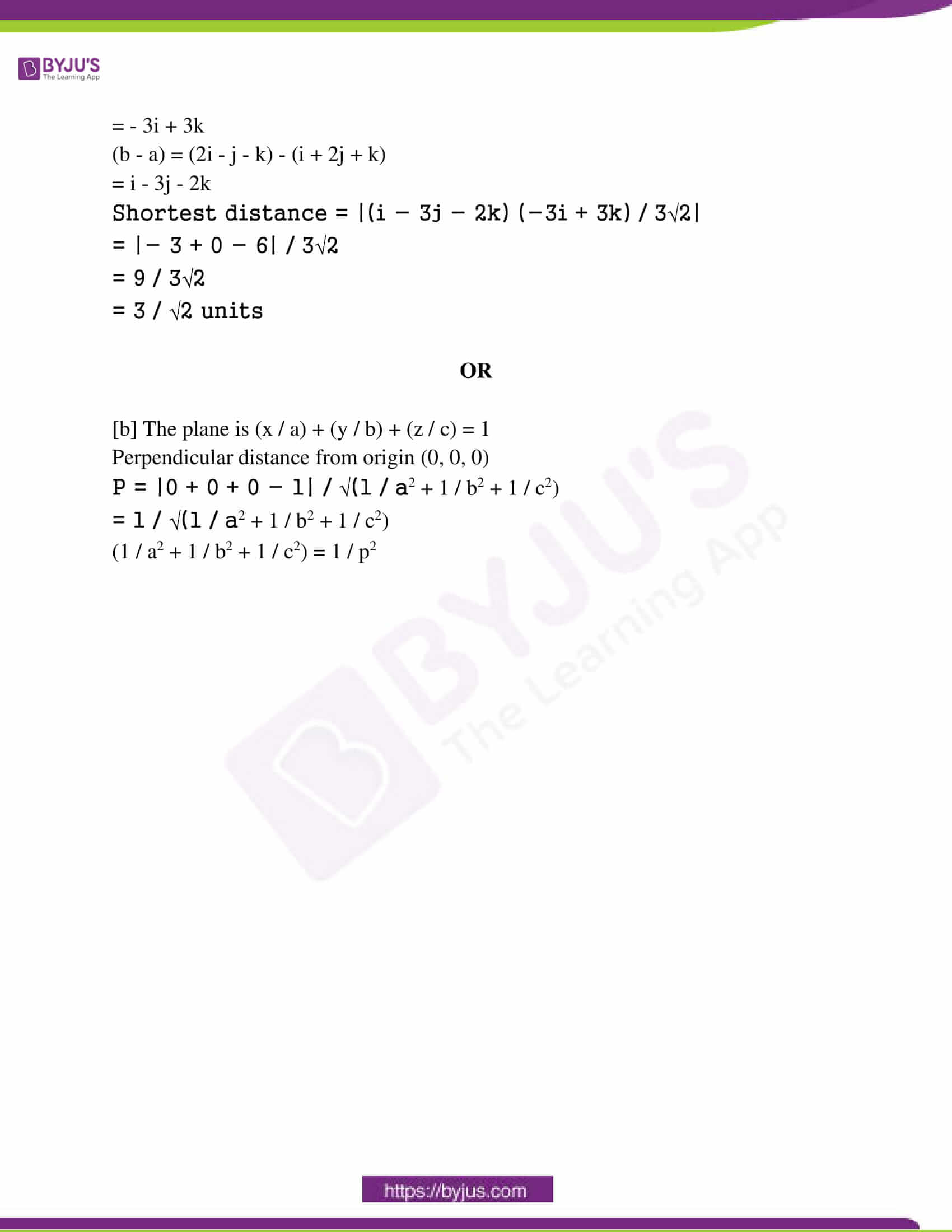SECTION – A

Question 1: Find x, if tan-1 3 + cot-1 x = 𝛑 / 2.

Solution:

tan-1 3 + cot-1 x = 𝛑 / 2

tan-1 3 = 𝛑 / 2 – cot-1 x

tan-1 x + cot-1 x = 𝛑 / 2

tan-1 x = 𝛑 / 2 – cot-1 x

tan-1 3 = tan-1 x

x = 3

Question 2: Construct a 2 × 2 matrix A = [aij], whose elements are given by aij = |– 5i + 2j|.

Solution:

aij = |– 5i + 2j|

The matrix A of order 2 × 2 is given by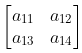.

a11 = |- 5 + 2| = 3

a12 = |- 5 + 4| = 1

a21 = |-10 + 2| = 8

a22 = |-10 + 4| = 6

A =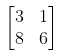Question 3: If [x -3]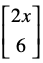= 0, then find the value of x.

Solution:

[x -3]= 0

[2x2 – 18] = 0

2x2 – 18 = 0

2x2 = 18

x2 = 18 / 2

x2 = 9

x = ± 3

Question 4: Find ∫[tan x / cot x] dx.

Solution:

∫[tan x / cot x] dx

tan x = 1 / cot x

= ∫tan2 x dx

= ∫sec2 x dx – ∫1 dx

= tan x – x + c

Question 5: Find the general solution of the differential equation (dy / dx) – (y / x) = 0.

Solution:

(dy / dx) – (y / x) = 0

(dy / dx) = (y / x)

∫dy / y = ∫dx / x

ln y = ln x + c

ln y = ln xc

y = xc

Question 6: If a = 2i – j + 5k and b = 4i – 2j + ƛk such that a || b, find the value of λ.

Solution:

a || b

a = kb

(2i – j + 5k) = k (4i – 2j + ƛk)

2 = 4k

– 1 = -2

5k = ƛk

k = 1 / 2

5 = (1 / 2)ƛ

ƛ = 10

Question 7: Find the direction cosine of the line x / 4 = y / 7 = z / 4.

Solution:

The direction ratios of the line are x / 4 = y / 7 = z / 4.

The direction cosine of the line are x / 4 = y / 7 = z / 4 are 4 / √42 + 72 + 42, 7 / √42 + 72 + 42, 4 / √42 + 72 + 42.

= 4 / 9, 7 / 9, 4 / 9

Question 8: Find the angle between planes r . (i – j + k) = 5 and r . (2i + j – k) = 7.

Solution:

Let the normal of the planes be a = i – j + k and b = 2i + j – k.

a . b = |a| . |b| cos θ

cos θ = (a . b) / |a| . |b|

cos θ = [i – j + k] [2i + j – k] / |i – j + k| |2i + j – k|

= [2 – 1 – 1] / √1 + 1 + 1 √4 + 1 + 1

cos θ = 0

θ = cos-1 0

θ = 𝛑 / 2

Question 9: Show the region of the feasible solution under the following constraints 2x + y ≥ 8, x ≥ 0, y ≥ 0 in the answer book.

Solution: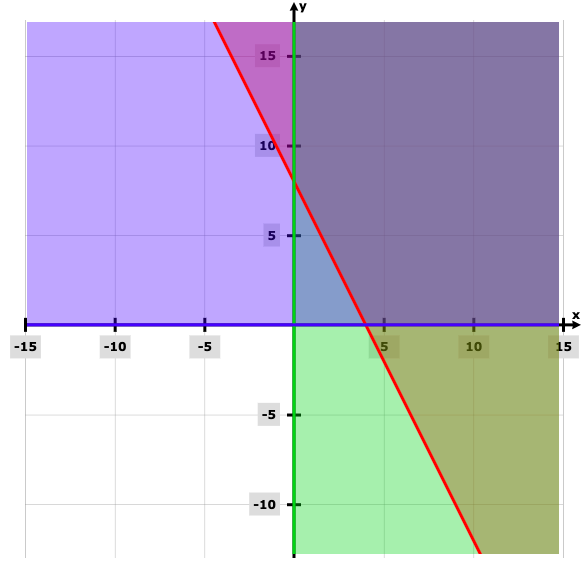Question 10: If A and B are independent events with P (A) = 0.2 and P (B) = 0.5, then find the value of P (A ∪ B).

Solution:

𝑃 (𝐴 ∪ 𝐵) = 𝑃 (𝐴) + 𝑃 (𝐵) − 𝑃 (𝐴 ∩ 𝐵)

𝑃 (𝐴 ∪ 𝐵) = 𝑃 (𝐴) + 𝑃 (𝐵) − 𝑃 (𝐴) × 𝑃 (𝐵)

= (0.2) + (0.5) – (0.2) (0.5)

= 0.7 – 0.10

= 0.6

SECTION – B

Question 11: [a] Prove that the relation R in a set of real numbers R defined as R = {(a, b) : a ≥ b} is reflexive and transitive but not symmetric.

OR

[b] Consider f : R → R given by f (x) = 2x + 3. Show that f is invertible. Find also the inverse of function f.

Solution:

[a] R = {(a, b) : a ≥ b}

Reflexive

R = {(a, a) : a > a}

Hence, R is reflexive.

Symmetric

Let (a, b) ∈ R

aRb ⇔ a ≥ b

bRa ⇔ b ≥ a not true

(b, a) ∉ R

Hence, R is not symmetric.

Transitive

Let (a, b) ∈ R and (b, c) ∈ R

aRb ⇔ a ≥ b

bRc ⇔ b ≥ c

a ≥ c

(a, c) ∈ R

Hence, R is transitive.

So, R is reflexive and transitive but not symmetric.

OR

[b] f (x) = 2x + 3

f (x1) = f (x2)

2x1 + 3 = 2x2 + 3

x1 = x2

Therefore, f (x) is a one-one function.

In f (x) = 2x + 3, the domain of x and y are R, hence the domain and co-domain are the same.

Therefore, f (x) is onto function.

So, f (x) is a one-one and onto function.

Hence, the inverse of f (x) exists.

Let f (x) = y

y = 2x + 3

2x = y – 3

x = (y – 3) / 2

Replacing x by y and y by x,

y = (x – 3) / 2

f -1 (x) = (x – 3) / 2

Question 12: [a] Prove that tan-1 (2 / 9) + tan-1 (1 / 4) = (1 / 2) sin-1 (4 / 5)

OR

[b] Solve 2 tan-1 (sin x) = tan-1 (2 sec x), 0 < x < 𝛑 / 2.

Solution:

[a] LHS = tan-1 (2 / 9) + tan-1 (1 / 4)

tan-1 x + tan-1 y = tan-1 [(x + y) / (1 – xy)]

= tan-1 [(2 / 9) + (1 / 4) / (1 – (2 / 9) (1 / 4)]

= tan-1 {[(8 + 9) / 36] / (1 – 2 / 36)}

= tan-1 [(17 / 36) / (34 / 36)]

= tan-1 (1 / 2)

= (1 / 2) sin-1 (2 * (1 / 2)) / (1 + (1 / 4))

= (1 / 2) sin-1 (4 / 5)

OR

[b] 2 tan-1 (sin x) = tan-1 (2 sec x)

2 tan-1 x = tan-1 (2x / (1 – x))

tan-1 [(2 sin x) / (1 – sin2 x)] = tan-1 [2 sec x]

(2 sin x) / (1 – sin2 x) = 2 sec x

sin x / cos2 x = sec x

sin x sec x = 1

sin x / cos x = 1

tan x = 1

x = 𝛑 / 4

Question 13: Express the matrix A =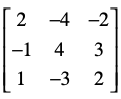as the sum of a symmetric and a skew-symmetric matrix.

Solution:

A = [(A + AT) / 2] + [(A – AT) / 2]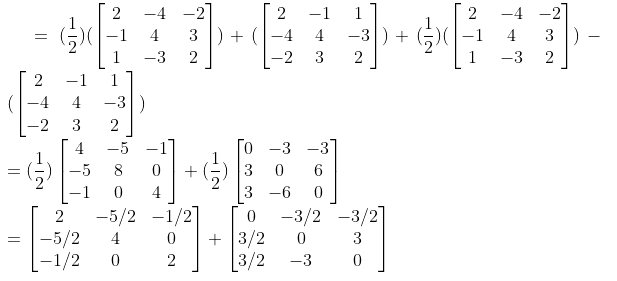Question 14: Find the value of K so that the function is continuous at the point x = 𝛑 / 2.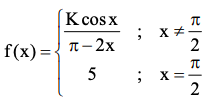Solution:Right hand limit at x = 𝛑 / 2

lim x→(𝛑 / 2)+ f (x) = lim h→0 f (𝛑 / 2 + h)

= lim h→0 [K cos (𝛑 / 2 + h)] / [(𝛑 – 2) (𝛑 / 2 + h)]

= lim h→0 – K sin h / – 2h

= (K / 2) lim h→0 sin h / h

= K / 2

For f (x) to be continuous, f (𝛑 / 2)+ = f (𝛑 / 2)

K / 2 = 5

K = 10

Question 15: [a] Find the intervals in which the function f given by f (x) = x2 – 6x + 5 is

i) Strictly increasing

ii) Strictly decreasing

OR

[b] Find the equation of the tangent to the curve x2/3 + y2/3 = 1 at the point (1, 1).

Solution:

[a] f (x) = x2 – 6x + 5

f ‘ (x) = 2x – 6

Put f ‘ (x) = 0

2x – 6 = 0

x = 3

Then divide the given interval into two parts as (-∞, 3) and (3, ∞).

[i] Take the interval (3, ∞)

f ‘ (x) = 2x – 6 > 0

The given function is strictly increasing in the interval (3, ∞).

[ii] Take the interval (-∞, 3)

f ‘ (x) = 2x – 6 < 0

The given function is strictly decreasing in the interval (-∞, 3).

OR

[b] x2/3 + y2/3 = 1

(2 / 3) x-⅓ + (2 / 3) y-⅓ (dy / dx) = 0

(1 / y) dy / dx = (- 1 / x)

dy / dx = – (y / x)

At (1, 1) dy / dx = -1

y – 1 = – 1 (x – 1)

y – 1 + x – 1 = 0

x + y – 2 = 0

Question 16: The radius of a circle is increasing uniformly at the rate of 5 cm/sec. Find the rate at which the area of the circle is increasing when the radius is 6 cm.

Solution:

Let the radius and area of the circle be r and A.

dr / dt = 5 cm/sec

A = 𝛑r2

dA / dt = 2𝛑r (dr / dt)

= 2𝛑 * 6 * 5

= 60𝛑 cm2/sec

Question 17: [a] Find ∫[(x – 1) (x – log x)3 / x] dx

OR

[b] Find ∫log (x2 + 1) dx.

Solution:

[a] ∫[(x – 1) (x – log x)3 / x] dx

Put x – log x = t

(1 – (1 / x)) dx = dt

([x – 1] / x) dx = dt

∫[(x – 1) (x – log x)3 / x] dx = ∫t3 dt

= t4 / 4 + c

= (x – log x)4 / 4 + c

OR

[b] ∫log (x2 + 1) dx

= ∫1 log (x2 + 1) dx

= log (x2 + 1) ∫dx – ∫[(d / dx) (log (x2 + 1)) ∫dx] dx

= x log (x2 + 1) – ∫2x2 / (x2 + 1) dx

= x log (x2 + 1) – 2 [∫dx – ∫1 / x2 + 1 dx]

= x log (x2 + 1) – 2 [x – tan-1 x] + c

= x log (x2 + 1) – 2x + tan-1 x + c

Question 18: Find ∫1 / [3x2 + 6x + 2].

Solution:

∫1 / [3x2 + 6x + 2]

= (1 / 3) ∫[1 / x2 + 2x + 1 + (2 / 3) – 1] dx

= (1 / 3) ∫[1 / x2 + 2x + 1 – (1 / 3)]

= (1 / 3) ∫1 / (x + 1)2 – (1 / √3)2

= (1 / 3) / 2 (1 / √3) log |(x + 1 – (1 / √3)) / (x + 1 – (1 / √3))| + c

= (1 / 2√3) log |(x + 1 – (1 / √3)) / (x + 1 – (1 / √3))| + c

Question 19: Find the area bounded by the parabola y2 = 4x and the straight line y = x. (Draw the figure in answer book)

Solution: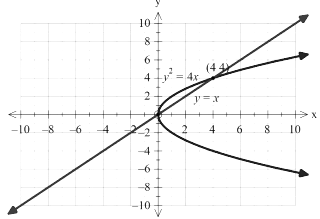y2 = 4x and y = x

y2 = 4y

y2 – 4y = 0

y (y – 4) = 0

y = 0, 4

Area = ∫04 (2√x – x) dx

= [(4 / 3) x3/2 – (x2 / 2)]04

= [(4 / 3) (4)3/2 – 8 – 0]

= (32 / 3) – 8

= 8 / 3 square units

Question 20: Using integration, find the area of a triangular region whose sides have the equations y = x + 1, y = 2x + 1 and x = 2. (Draw the figure in answer book)

Solution:

Consider y = x + 1 and y = 2x + 1

Equate both the equations and solve for x

x + 1 = 2x + 1

x = 0

Substitute x = 0 in y = x + 1,

y = 1

Substitute x = 2 in y = 2x + 1

y = 5

Graph of y = x + 1, y = 2x + 1 and x = 2 is given by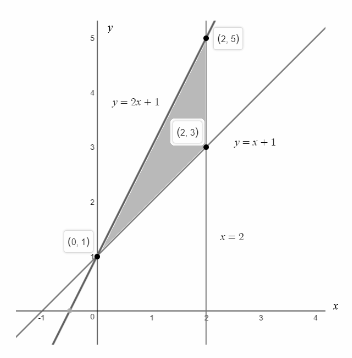Area = ∫02 [2x + 1 – x – 1] dx

= ∫02 x dx

= [x2 / 2]02

= 2 square units

Question 21: If a, b, c are unit vectors such that a + b + c = 0, find the value of a . b + b . c + c . a.

Solution:

a + b + c = 0

(a + b + c)2 = 0

|a|2 + |b|2 + |c|2 + 2 (a . b + b . c + c . a) = 0

1 + 1 + 1 + 2 (a . b + b . c + c . a) = 0

2 (a . b + b . c + c . a) = – 3

(a . b + b . c + c . a) = -3 / 2

Question 22: Find a unit vector perpendicular to each of the vectors 2a + b and a – 2b, where a = i + 2j – k, b = i + j + k.

Solution:

a = i + 2j – k

b = i + j + k

2a + b = 2 (i + 2j – k) + i + j + k

= 3i + 5j – k

a – 2b = (i + 2j – k) – 2 (i + j + k)

= – i – 3k

The vector perpendicular to both 2a + b and a – 2b is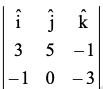= -15i + 10j + 5k

Unit vector is given by

= [-15i + 10j + 5k] / √225 + 100 + 25

= [-15i + 10j + 5k] / √350

= [-15i + 10j + 5k] / 5√14

= [-3i + 2j + k] / √14

Question 23: By graphical method solve the following linear programming problem for maximization.

Objective function Z = 1000x + 600y

Constraints

x + y ≤ 200

4x – y ≤ 0

x ≥ 20, x ≥ 0, y ≥ 0.

Solution: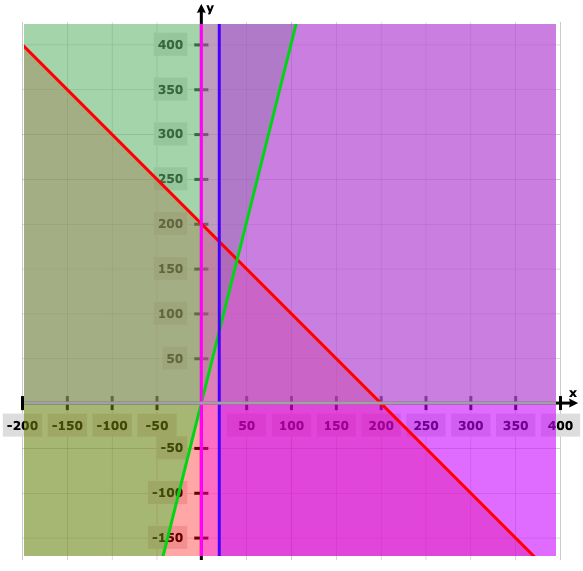Points Z (20, 80) 68000 (20, 180) 128000 (40, 160) 136000

The maximum value of Z = 1000x+ 600y is 136000 at x = 40 and y =160.

Question 24: Bag A contains 2 red and 3 black balls while another bag B contains 3 red and 4 black balls. One ball is drawn at random from one of the bags and it is found to be red. Find the probability that it was drawn from bag B.

Solution:

Consider the events E and F as follows:

E: Bag A is selected

F: Bag B is selected

A: Ball drawn is red

Since there are only two bags.

Bag A contains 2 red and 3 black balls

P (A / E) = 2 / 5

Bag B contains 3 red and 4 black balls

P (A / F) = 3 / 7

The required probability is

P (F / A) = [P (F) P (A / F)] / [P (E) P (A / E) + P (F) P (A / F)]

= (1 / 2) (3 / 7) / [(1 / 2) * (2 / 5) + (1 / 2) * (3 / 7)]

= (8 / 35) / [(3 / 14) + (8 / 35)]

= (3 / 14) / (29 / 70)

= 15 / 29

Question 25: From a lot of 30 bulbs which include 6 defectives, a sample of 2 bulbs is drawn at random with replacement. Find the probability distribution of the number of defective bulbs.

Solution:

Let X be a random variable.

X = number of defective bulbs.

Clearly, X can assume the value 0, 1 or 2.

P (X = 0) = 24C2 / 30C2 = 92 / 145

P (X = 1) = 6C1 * 24C1 / 30C2 = 48 / 145

P (X = 2) = 6C2 / 30C2 = 1 / 129

SECTION – C

Question 26: Prove that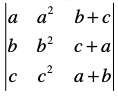= (a + b + c) (a – b) (b – c) (c – a).

Solution: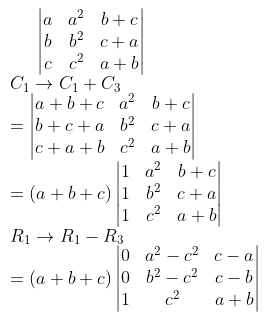= (a + b + c) [(a2 – c 2) (c – b) – (c – a) (b2 – c2)]

= (a + b + c) [(a – c) (a + c) (c – b) – (c – a) (b – c) (b + c)]

= (a + b + c) (b – c) (c – a) [(a + c) – (b + c)]

= (a + b + c) (a – b) (b – c) (c – a)

Question 27: If y = [sin-1 x]2 then show that (1 – x2) (d2y / dx2) – x (dy / dx) – 2 = 0.

Solution:

y = [sin-1 x]2

dy / dx = 2 sin-1 x / √1 – x2 —– (1)

d2y / dx2 = {(2 √1 – x2) * (1 / √1 – x2) – (2 sin-1 x) * (1 / 2) * (-2x / √1 – x2)} / (1 – x2)

(1 – x2) (d2y / dx2) = 2 + x (2 sin-1 x) / √1 – x2)

(1 – x2) (d2y / dx2) = 2 + x (dy / dx)

(1 – x2) (d2y / dx2) – x (dy / dx) – 2 = 0

Question 28: Evaluate ∫0𝛑 [x sinx / 1 + cos2 x] dx.

Solution:

I = ∫0𝛑 [x sinx / 1 + cos2 x] dx —- (1)

= ∫0𝛑 [(𝛑 – x) sin (𝛑 – x) / 1 + cos2 (𝛑 – x)] dx

= ∫0𝛑 [(𝛑 – x) sin x / 1 + cos2 x] dx —- (2)

2I = 𝛑 ∫0𝛑 sin x / (1 + cos2 x) dx

2I = 2𝛑 ∫0𝛑/2 (sin x dx) / (1 + cos2 x)

I = 𝛑 ∫0𝛑/2 (sin x dx) / (1 + cos2 x)

Let cos x = t

– sinx dx = dt

I = 𝛑 ∫10 – dt / 1 + t2

I = – 𝛑 [tan-1 t]10

I = – 𝛑 [tan-1 0 – tan-1 1]

= – 𝛑 [- 𝛑 / 4]

= 𝛑2 / 4

Question 29: [a] Solve the differential equation 2xy + y2 – 2x2 (dy / dx) = 0

OR

[b] Solve the differential equation dy / dx + y cot x = 2x + x2 cot x.

Solution:

[a] 2xy + y2 – 2x2 (dy / dx) = 0

2x2 (dy / dx) = 2xy + y2

dy / dx = (y / x) + (1 / 2) (y / x)2 —– (1)

Ley y = vx

dy / dx = v + x (dv / dx)

Put in (1)

v + x (dv / dx) = v + (1 / 2)v2

x (dv / dx) = v2 / 2

dv / v2 = dx / 2x

On integrating both sides,

∫dv / v2 = ∫dx / 2x

(- 1 / v) = (1 / 2) log x + c

(- x / y) = (1 / 2) log x + c

OR

[b] dy / dx + y cot x = 2x + x2 cot x

This is a linear differential equation of the form (dy / dx) + Py = Q

P = cot x, Q = 2x + x2 cot x

IF = e∫P dx = e∫cot x dx = elog sinx = sin x

Multiply both sides by I.F. & then integrate

y sin x = ∫2x sin x dx + ∫x2 cot x sin x dx

y sin x = 2 ∫x sin x dx + ∫x2 cos x dx

= 2 ∫x sin x dx + x2 sin x – ∫2x sin x dx + c

y sin x = x2 sin x + c

Question 30: [a] Find the shortest distance between the lines (x – 1) / 1 = (y – 2) / -1 = (z – 1) / 1 and (x – 2) / 2 = (y + 1) / 1 = (z + 1) / 2.

OR

[b] Prove that if a plane has the intercepts a, b, c and is at distance p units from the origin, then prove that 1 / a2 + 1 / b2 + 1 / c2 = 1 / p2.

Solution:

[a] (x – 1) / 1 = (y – 2) / -1 = (z – 1) / 1

⇒ (r – [i + 2j + k]) . (i – j + k) = 0

(x – 2) / 2 = (y + 1) / 1 = (z + 1) / 2

⇒ (r – [2i – j – k]) . (2i + j + 2k) = 0

Shortest distance = |[(b – a) . (p x q)] / [p x q]|

[p x q] =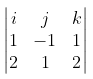= i (- 2 – 1) – j (2 – 2) + k (1 + 2)

= – 3i + 3k

(b – a) = (2i – j – k) – (i + 2j + k)

= i – 3j – 2k

Shortest distance = |(i – 3j – 2k) (-3i + 3k) / 3√2|

= |- 3 + 0 – 6| / 3√2

= 9 / 3√2

= 3 / √2 units

OR

[b] The plane is (x / a) + (y / b) + (z / c) = 1

Perpendicular distance from origin (0, 0, 0)

P = |0 + 0 + 0 – 1| / √(1 / a2 + 1 / b2 + 1 / c2)

= 1 / √(1 / a2 + 1 / b2 + 1 / c2)

(1 / a2 + 1 / b2 + 1 / c2) = 1 / p2